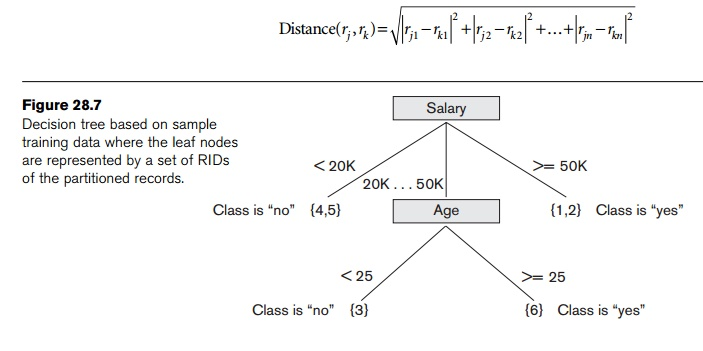# Clustering

1. Market-Basket Model, Support, and Confidence 2. Apriori Algorithm 3. Sampling Algorithm 4. Frequent-Pattern (FP) Tree and FP-Growth Algorithm 5. Partition Algorithm 6. Other Types of Association Rules 7. Additional Considerations for Association Rules

Clustering

The previous data mining task of classification deals with partitioning data based on using a preclassified training sample. However, it is often useful to partition data without having a training sample; this is also known as unsupervised learning. For example, in business, it may be important to determine groups of customers who have similar buying patterns, or in medicine, it may be important to determine groups of patients who show similar reactions to prescribed drugs. The goal of clustering is to place records into groups, such that records in a group are similar to each other and dissimilar to records in other groups. The groups are usually disjoint.

An important facet of clustering is the similarity function that is used. When the data is numeric, a similarity function based on distance is typically used. For exam-ple, the Euclidean distance can be used to measure similarity. Consider two n-dimensional data points (records) rj and rk. We can consider the value for the ith dimension as rji and rki for the two records. The Euclidean distance between points rj and rk in n-dimensional space is calculated as:The smaller the distance between two points, the greater is the similarity as we think of them. A classic clustering algorithm is the k-Means algorithm, Algorithm 28.4.

Algorithm 28.4. k-Means Clustering Algorithm

Input: a database D, of m records, r1, ..., rm and a desired number of clusters k

Output: set of k clusters that minimizes the squared error criterion

Begin

randomly choose k records as the centroids for the k clusters; repeat assign each record, ri, to a cluster such that the distance betweenrand the cluster centroid (mean) is the smallest among the k clusters; recalculate the centroid (mean) for each cluster based on the records assigned to the cluster; until no change;

End;

The algorithm begins by randomly choosing k records to represent the centroids (means), m1, ..., mk, of the clusters, C1, ..., Ck. All the records are placed in a given cluster based on the distance between the record and the cluster mean. If the distance between mi and record rj is the smallest among all cluster means, then record rj is placed in cluster Ci. Once all records have been initially placed in a cluster, the mean for each cluster is recomputed. Then the process repeats, by examining each record again and placing it in the cluster whose mean is closest. Several iterations may be needed, but the algorithm will converge, although it may terminate at a local optimum. The terminating condition is usually the squared-error criterion. For clusters C1, ..., Ck with means m1, ..., mk, the error is defined as:We will examine how Algorithm 28.4 works with the (two-dimensional) records in Figure 28.8. Assume that the number of desired clusters k is 2. Let the algorithm choose records with RID 3 for cluster C1 and RID 6 for cluster C2 as the initial cluster centroids. The remaining records will be assigned to one of those clusters during thefirst iteration of the repeat loop. The record with RID 1 has a distance from C1 of 22.4 and a distance from C2 of 32.0, so it joins cluster C1. The record with RID 2 has a distance from C1 of 10.0 and a distance from C2 of 5.0, so it joins cluster C2. The record withRID 4 has a distance from C1 of 25.5 and a distance from C2 of 36.6, so it joins cluster C1. The record with RID 5 has a distance fromC1 of 20.6 and a dis-tance from C2 of 29.2, so it joins cluster C1. Now, the new means (centroids) for the two clusters are computed. The mean for a cluster, Ci, with n records of m dimensions is the vector:The new mean for C1 is (33.75, 8.75) and the new mean for C2 is (52.5, 25). A second iteration proceeds and the six records are placed into the two clusters as follows: records with RIDs 1, 4, 5 are placed in C1 and records with RIDs 2, 3, 6 are placed in C2. The mean for C1 and C2 is recomputed as (28.3, 6.7) and (51.7, 21.7), respectively. In the next iteration, all records stay in their previous clusters and the algo-rithm terminates.

Traditionally, clustering algorithms assume that the entire data set fits in main memory. More recently, researchers have developed algorithms that are efficient and are scalable for very large databases. One such algorithm is called BIRCH. BIRCH is a hybrid approach that uses both a hierarchical clustering approach, which builds a tree representation of the data, as well as additional clustering methods, which are applied to the leaf nodes of the tree. Two input parameters are used by the BIRCH algorithm. One specifies the amount of available main memory and the other is an initial threshold for the radius of any cluster. Main memory is used to store descriptive cluster information such as the center (mean) of a cluster and the radius of the cluster (clusters are assumed to be spherical in shape). The radius threshold affects the number of clusters that are produced. For example, if the radius threshold value is large, then few clusters of many records will be formed. The algorithm tries to maintain the number of clusters such that their radius is below the radius threshold. If available memory is insufficient, then the radius threshold is increased.

The BIRCH algorithm reads the data records sequentially and inserts them into an in-memory tree structure, which tries to preserve the clustering structure of the data. The records are inserted into the appropriate leaf nodes (potential clusters) based on the distance between the record and the cluster center. The leaf node where the insertion happens may have to split, depending upon the updated center and radius of the cluster and the radius threshold parameter. Additionally, when splitting, extra cluster information is stored, and if memory becomes insufficient, then the radius threshold will be increased. Increasing the radius threshold may actually produce a side effect of reducing the number of clusters since some nodes may be merged.

Overall, BIRCH is an efficient clustering method with a linear computational complexity in terms of the number of records to be clustered.

Study Material, Lecturing Notes, Assignment, Reference, Wiki description explanation, brief detail
Fundamentals of Database Systems : Advanced Database Models, Systems, and Applications : Data Mining Concepts : Clustering |